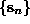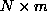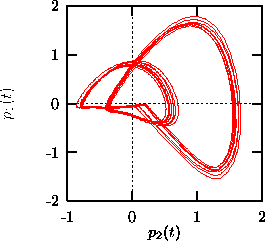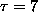Next: Poincaré sections Up: Phase space representation Previous: False nearest neighbors

## Principal components

It has been shown in Ref.  that the embedding technique can be generalized to a wide class of smooth transformations applied to a time delay embedding. In particular, if we introduce time delay coordinates, then almost every linear transformation of sufficient rank again leads to an embedding. A specific choice of linear transformation is known as principal component analysis, singular value decomposition, empirical orthogonal functions, Karhunen-Loéve decomposition, and probably a few other names. The technique is fairly widely used, for example to reduce multivariate data to a few major modes. There is a large literature, including textbooks like that by Jolliffe . In the context of nonlinear signal processing, the technique has been advocated among others by Broomhead and King .

The idea is to introduce a new set of orthonormal basis vectors in embedding space such that projections onto a given number of these directions preserve the maximal fraction of the variance of the original vectors. In other words, the error in making the projection is minimized for a given number of directions. Solving this minimization problem  leads to an eigenvalue problem. The desired principal directions can be obtained as the eigenvectors of the symmetric autocovariance matrix that correspond to the largest eigenvalues. The alternative and formally equivalent approach via the trajectory matrix is used in Ref. . The latter is numerically more stable but involves the singular value decomposition of anmatrix for N data points embedded in m dimensions, which can easily exceed computational resources for time series of even moderate length .

In almost all the algorithms described below, simple time delay embeddings can be substituted by principal components. In the TISEAN project (routines svd, pc), principal components are only provided as a stand-alone visualization tool and for linear filtering , see Sec.below. In any case, one first has to choose an initial time delay embedding and then a number of principal components to be kept. For the purpose of visualization, the latter is immediately restricted to two or at most three. In order to take advantage of the noise averaging effect of the principal component scheme, it is advisable to choose a much shorter delay than one would for an ordinary time delay embedding, while at the same time increasing the embedding dimension. Experimentation is recommended. Figureshows the contributions of the first two principal components to the magneto-cardiogram shown in Fig..Figure:   Phase space representation of a human magneto-cardiogram using the two largest principal components. An initial embedding was chosen in m=20 dimensions with a delay ofms. The two components cover 70% of the variance of the initial embedding vectors.Next: Poincaré sections Up: Phase space representation Previous: False nearest neighbors

Thomas Schreiber
Wed Jan 6 15:38:27 CET 1999